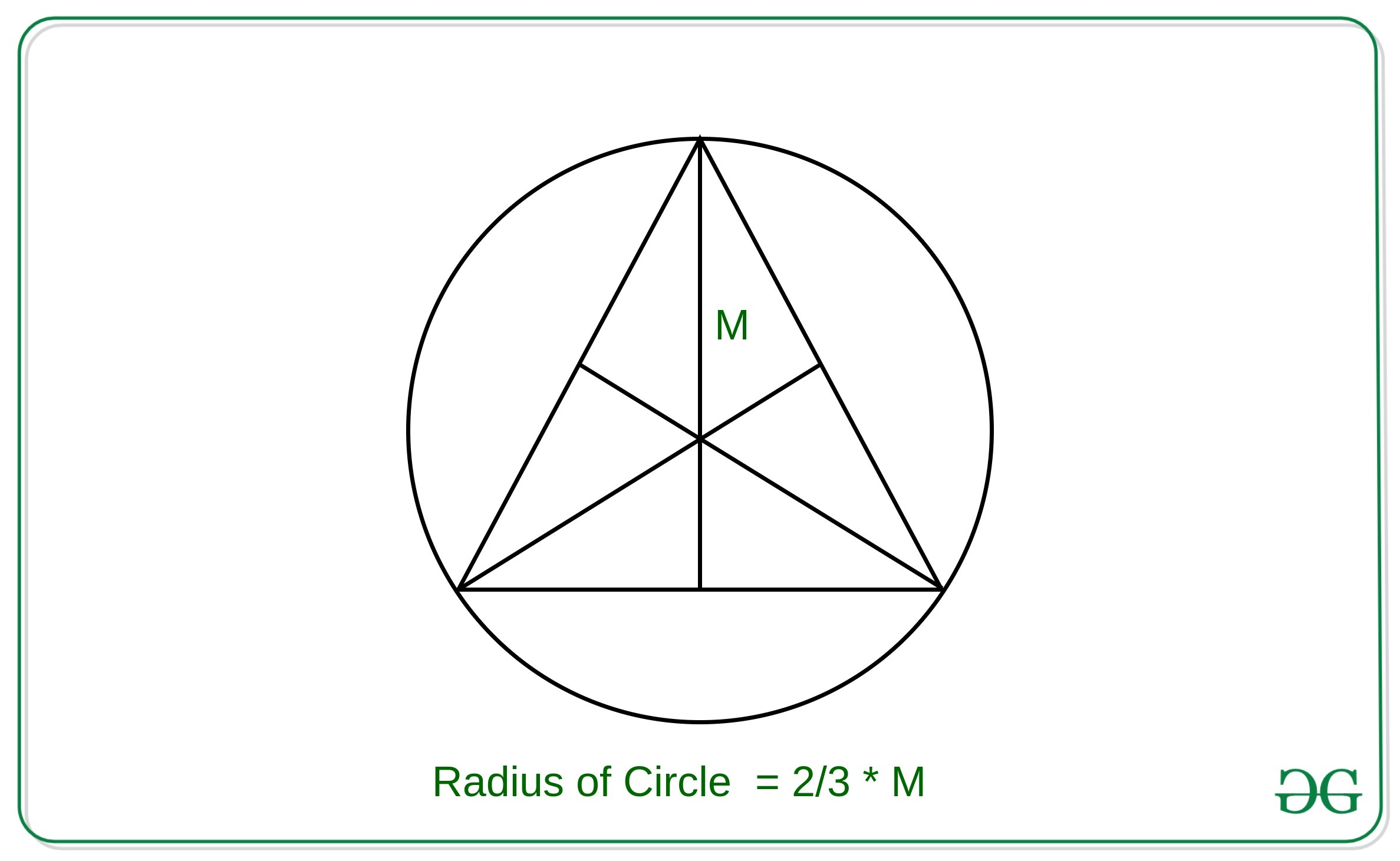# Area of Circumcircle of an Equilateral Triangle using Median

• Last Updated : 25 Mar, 2021

Given the median of the Equilateral triangle M, the task is to find the area of the circumcircle of this equilateral triangle using the median M.
Examples:

Input: M = 3
Output: 12.5664
Input: M = 6
Output: 50.2655

Attention reader! All those who say programming isn't for kids, just haven't met the right mentors yet. Join the  Demo Class for First Step to Coding Coursespecifically designed for students of class 8 to 12.

The students will get to learn more about the world of programming in these free classes which will definitely help them in making a wise career choice in the future.

Approach: The key observation in the problem is that the centroid, circumcenter, orthocenter and incenter of an equilateral triangle all lie at the same point.Therefore, the radius of the circle with the given median of the equilateral triangle inscribed in the circle can be derived as:Then the area of the circle can be calculated using the approach used in this article
Below is the implementation of the above approach:

## C++

 // C++ implementation to find the// equation of circle which// inscribes equilateral triangle// of median M #include const double pi = 3.14159265358979323846;using namespace std; // Function to find the equation// of circle whose center is (x1, y1)// and the radius of circle is rvoid circleArea(double r){    cout << (pi * r * r);} // Function to find the// equation of circle which// inscribes equilateral triangle// of median Mvoid findCircleAreaByMedian(double m){    double r = 2 * m / 3;     // Util Function to find the    // circle equation    circleArea(r);} // Driver codeint main(){    double m = 3;     // Function Call    findCircleAreaByMedian(m);    return 0;}

## Java

 // Java implementation to find the// equation of circle which// inscribes equilateral triangle// of median Mimport java.util.*; class GFG{     // Function to find the equation// of circle whose center is (x1, y1)// and the radius of circle is rstatic double circleArea(double r){    double pi = 3.14159265358979323846;    return (pi * r * r);}     // Function to find the// equation of circle which// inscribes equilateral triangle// of median Mstatic double findCircleAreaByMedian(int m){    double r = 2 * m / 3;         // Function call to find    // the circle equation    return circleArea(r);} // Driver codepublic static void main(String args[]){    int m = 3;         System.out.printf("%.4f", findCircleAreaByMedian(m));}} // This code is contributed by virusbuddah_

## Python3

 # Python3 implementation to find the# equation of circle which inscribes# equilateral triangle of median M pi = 3.14159265358979323846 # Function to find the equation# of circle whose center is (x1, y1)# and the radius of circle is rdef circleArea(r):         print(round(pi * r * r, 4)) # Function to find the# equation of circle which# inscribes equilateral triangle# of median Mdef findCircleAreaByMedian(m):         r = 2 * m /3     # Function to find the    # circle equation    circleArea(r) # Driver codeif __name__ == '__main__':         m = 3     # Function call    findCircleAreaByMedian(m) # This code is contributed by mohit kumar 29

## C#

 // C# implementation to find the// equation of circle which// inscribes equilateral triangle// of median Musing System; class GFG{     // Function to find the equation// of circle whose center is (x1, y1)// and the radius of circle is rstatic double circleArea(double r){    double pi = 3.14159265358979323846;    return (pi * r * r);}         // Function to find the// equation of circle which// inscribes equilateral triangle// of median Mstatic double findCircleAreaByMedian(int m){    double r = 2 * m / 3;             // Function call to find    // the circle equation    return circleArea(r);}     // Driver codepublic static void Main(string []args){    int m = 3;             Console.WriteLine("{0:f4}", findCircleAreaByMedian(m));}} // This code is contributed by AnkitRai01

## Javascript

 
Output:
12.5664

My Personal Notes arrow_drop_up